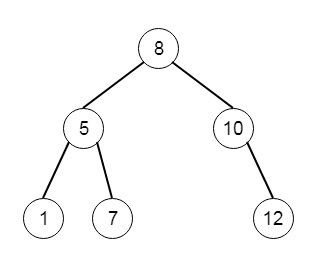# Construct Binary Search Tree from Preorder Traversal in Python

Suppose we have to create a binary search tree that matches the given preorder traversal. So if the pre-order traversal is like [8,5,1,7,10,12], then the output will be [8,5,10,1,7,null,12], so the tree will be −To solve this, we will follow these steps −

• root := 0th node of the preorder traversal list
• stack := a stack, and push root into the stack
• for each element i from the second element of the preorder list
• i := a node with value i
• if value of i < top of the stack top element, then
• left of stack top node := i
• insert i into the stack
• otherwise
• while stack is not empty, and stack top element value < value of i
• last := top of stack
• pop element from stack
• right of the last node := i
• insert i into the stack
• return root

Let us see the following implementation to get better understanding −

## Example

class Solution(object):
def bstFromPreorder(self, preorder):
"""
:type preorder: List[int]
:rtype: TreeNode
"""
root = TreeNode(preorder)
stack = [root]
for i in preorder[1:]:
i = TreeNode(i)
if i.val<stack[-1].val:
stack[-1].left = i
stack.append(i)
else:
while stack and stack[-1].val<i.val:
last = stack.pop(-1)
last.right = i
stack.append(i)
return root

## Input

[8,5,1,7,10,12]

## Output

[8,5,10,1,7,null,12]